# Intermediate Geometry : How to find out if a point is on a line with an equation

## Example Questions

1 3 Next →

### Example Question #21 : Other Lines

Which of the following points is on the line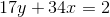?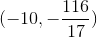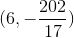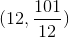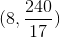Explanation:

Start by rewriting the equation into slope-intercept form.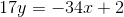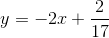To find which point is on the line, take the-coordinate, and plug it into the given equation to solve for. If the-value matches the-coordinate of the same point, then the point is on the line.

Plugging in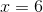into the given equation will give the following: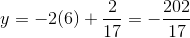Thus,is on the line.

### Example Question #22 : How To Find Out If A Point Is On A Line With An Equation

Which of the following points is on the line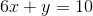?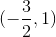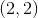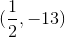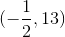Explanation:

Start by rewriting the equation into slope-intercept form.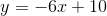To find which point is on the line, take the-coordinate, and plug it into the given equation to solve for. If the-value matches the-coordinate of the same point, then the point is on the line.

Plugging in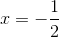into the given equation will give the following: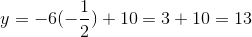Thus,is on the line.

### Example Question #23 : How To Find Out If A Point Is On A Line With An Equation

Which of the following points is found on the line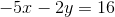?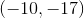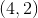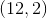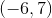Explanation:

Start by rewriting the equation into slope-intercept form.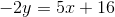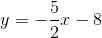To find which point is on the line, take the-coordinate, and plug it into the given equation to solve for. If the-value matches the-coordinate of the same point, then the point is on the line.

Plugging in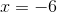into the given equation will give the following: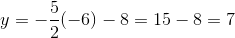Thus,is on the line.

### Example Question #24 : How To Find Out If A Point Is On A Line With An Equation

True or false:

The line of the equation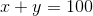passes through the point with coordinates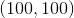.

True

False

False

Explanation:

A line of an equation passes through the point with coordinatesif and only if, when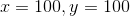, the equation is true. Substitute forand: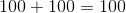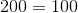- this is false.

The line does not pass through the point.

### Example Question #25 : How To Find Out If A Point Is On A Line With An Equation

True or false:

The line of the equation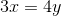passes through the origin.

False

True

True

Explanation:

The coordinates of the origin are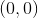, so the line of an equation passes through this point of and only ifis a solution of the equation - or, equivalently, if and only if settingand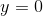makes the equation a true statement. Substitute both values: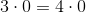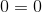The statement is true, so the line does pass through the origin.

### Example Question #26 : How To Find Out If A Point Is On A Line With An Equation

True or false:

The lines of the equations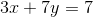and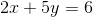intersect at the point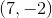.

(Note: You are given that the lines are distinct)

True

False

False

Explanation:

If two distinct lines intersect at the point- that is, if both pass through this point - it follows thatis a solution of the equations of both. Therefore, set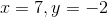in the equations and determine whether they are true or not.

Examine the second equation: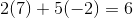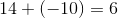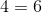False;is not on the line of this equation.

Therefore, the lines cannot intersect at.

1 3 Next →